# Solid Shapes

Go back to  'Geometry'

## Solid Shapes

Solid shapes are three dimensional shapes who have length, breadth and height as the three dimensions.

Here are a few solid shapes.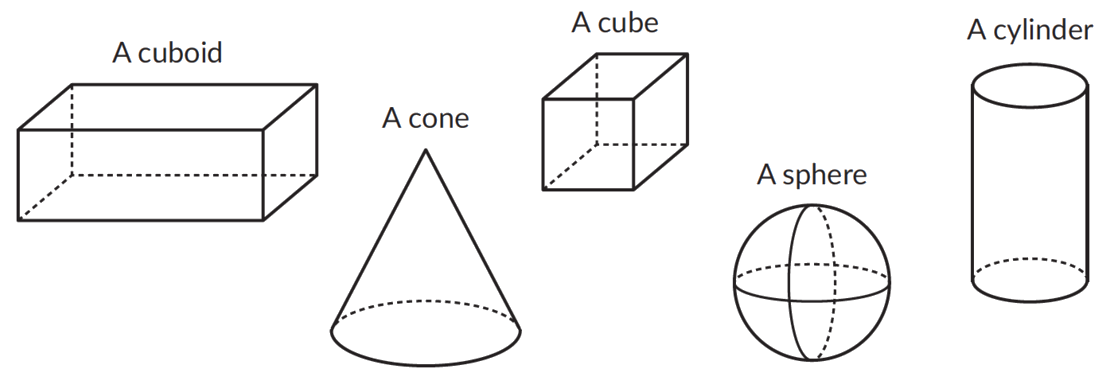### Solid shapes have faces, edges and vertices.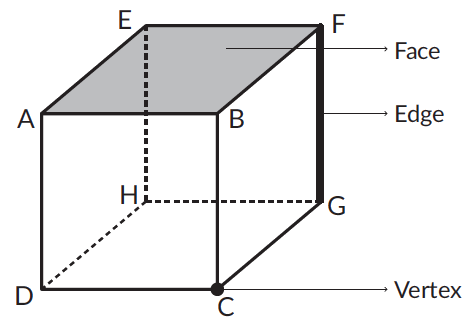## Polyhedron

A solid shape whose faces are all polygons is called a polyhedron.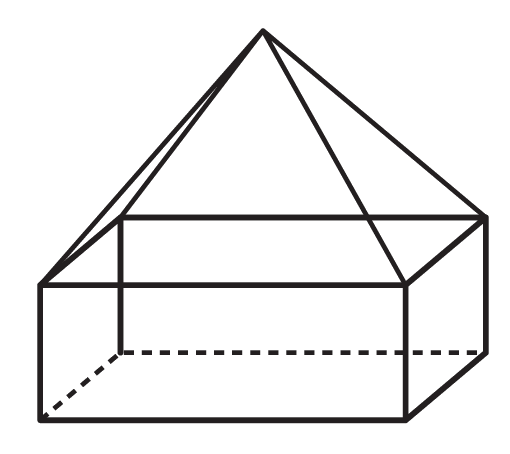### Pyramid

A pyramid is a polyhedron with: a polygon as its base and all other faces as triangles that meet at a point (Apex).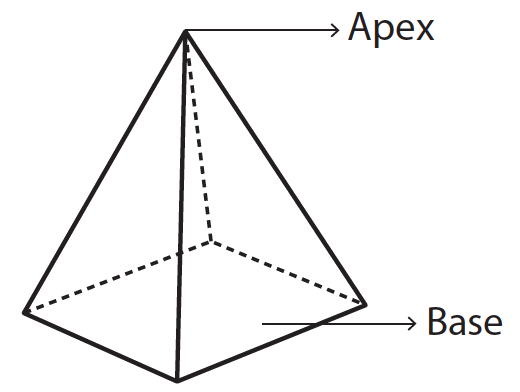Given below is a table showing the number of faces, edges, vertices for different polyhedrons.

 Solid Shapes Faces Edges Vertices Triangular pyramid (It's also called a Tetrahedron.)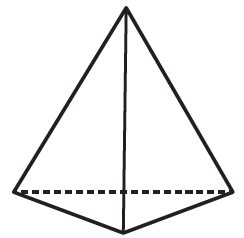4 6 4 Square Pyramid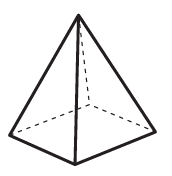5 8 5 Pentagonal Pyramid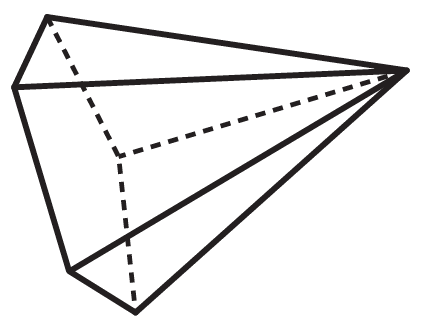6 10 6

### Prism

A prism is a polyhedron with two identical faces that are parallel and all other faces as parallelograms.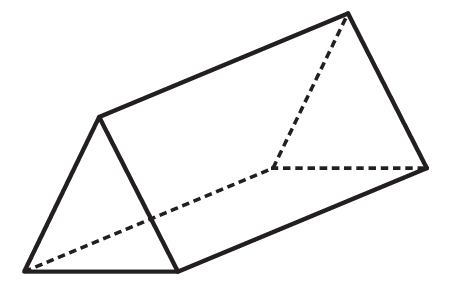Given below is a table showing the number of faces, edges, vertices for different types of prisms.

 Solid Shapes Faces Edges Vertices Triangular Prism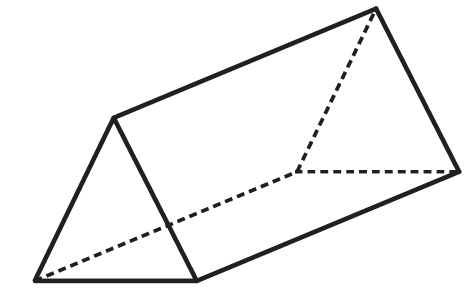5 9 6 Square Prism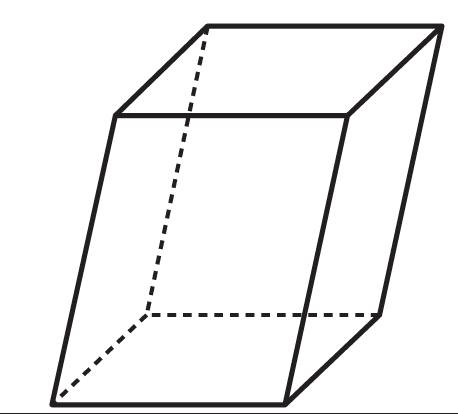6 12 8 Pentagonal Prism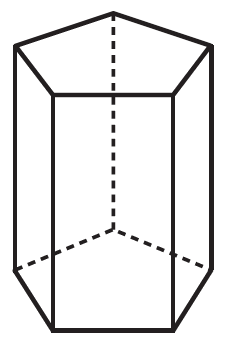7 15 10

### Formula - Euler’s formula

For every polyhedron, the value of $$F + V – E$$ is . This formula is called Euler’s formula

Let’s consider a few examples,

 Shape Faces Edges Vertices $$F + V – E$$5 8 5 5 + 5 - 8 = 2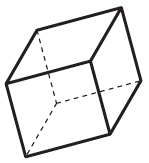6 12 8 6 + 8 - 12 = 2

## Tips and tricks

• Tip: Make these shapes using plain sheet of paper. This would help visualise the solid shapes.
• Tip: Using the geosolid, move your finger along each shape to understand the concept of faces, edges and vertices.
• Tip: Use a paper that has grid lines on it. The grid lines make it easy to see if the line of symmetry is dividing the figure into two identical parts.
• Tip: Draw a free hand design on an A4 sheet. Now fold the paper in to one half. If the figure is exactly symmetrical than one part should match the other.

### Common mistakes or misconceptions

Misconception 1: A line of symmetry is always vertical.
A line of symmetry can be vertical, horizontal or diagonal as long as it divides the figure into two identical parts.

1. Which is a pentagonal pyramid?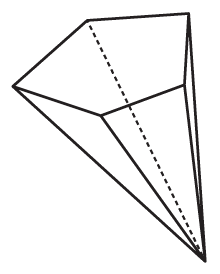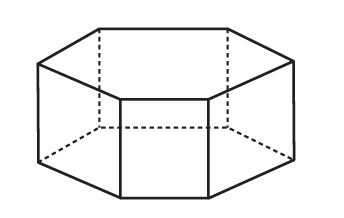2. Which is a pentagonal prism?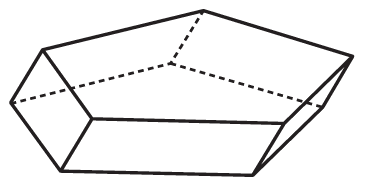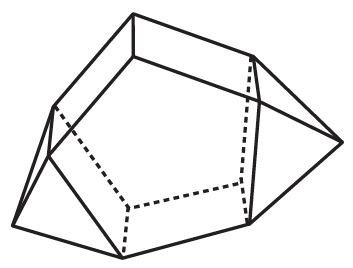3. Which is a hexagonal prism?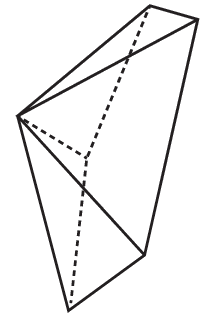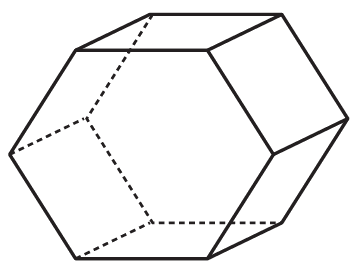4. Draw all the possible lines of symmetry for each letter of the English alphabet. Also write in the number of lines of symmetry.a. Number of lines of symmetry ________________________b. Number of lines of symmetry________________________c. Number of lines of symmetry________________________

5. Write yes or no if the first figure is the mirror image of the second figure, about the given line $$l.$$

a.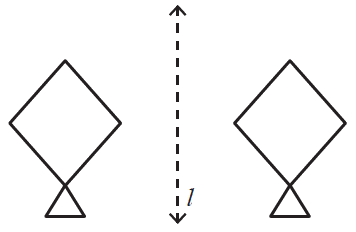b.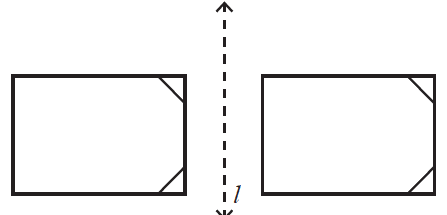## Important Topics

More Important Topics
Numbers
Algebra
Geometry
Measurement
Money
Data
Trigonometry
Calculus